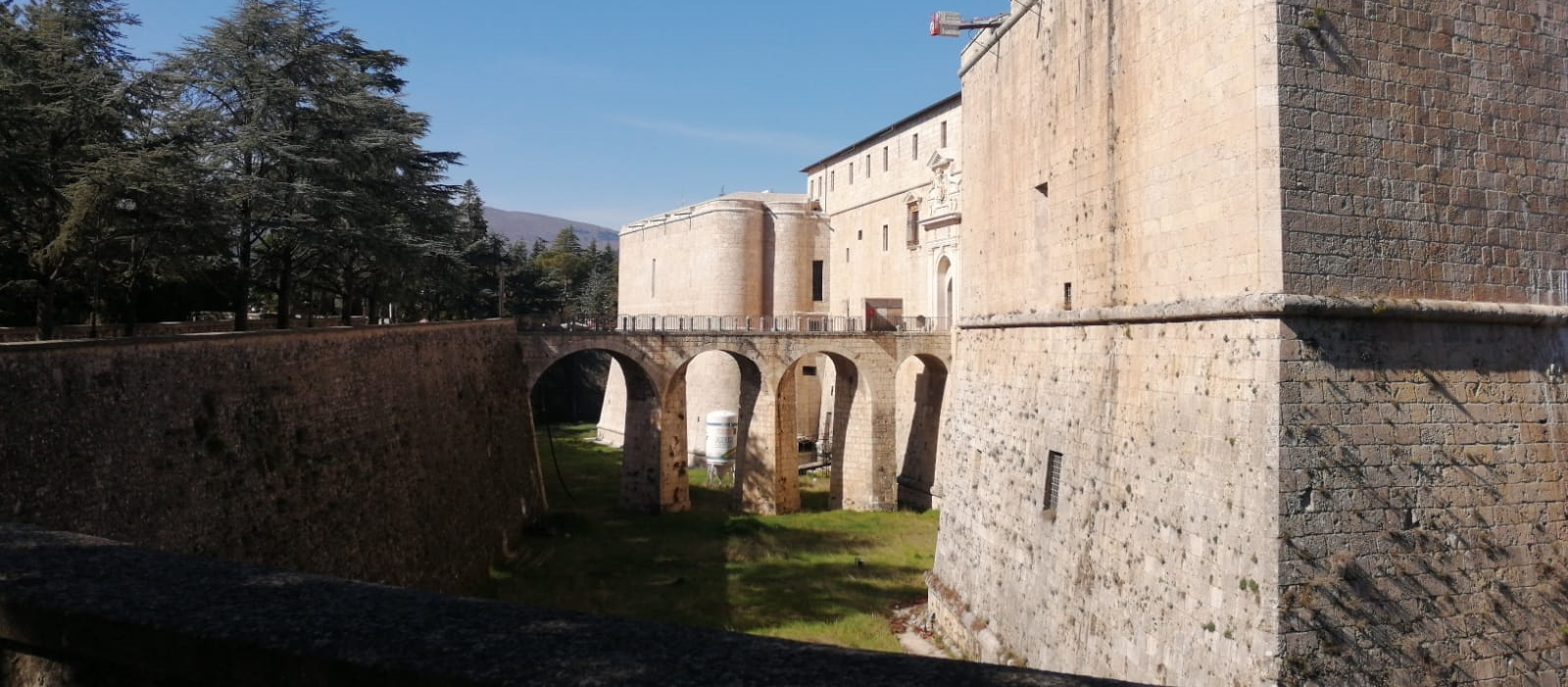# Trials in Wave Turbulence: from random waves to kinetic equations

Sep 5 – 10, 2022
Gran Sasso Science Institute, L'Aquila (IT)
Europe/Rome timezone

## Scientific DescriptionSpanish fortress, L'Aquila

Nonlinear interacting waves are ubiquitous in nature. When the range of wavelengths becomes very large, a statistical description of such waves and their interactions is fundamental. Wave Turbulence (WT) provides a mathematical framework to describe those phenomena, and as such it applies to a great variety of physical systems, like for instance quantum fluids (quantum vortex filaments, nonlinear waves in Bose-Einstein condensates and more generally quantum turbulence), water waves, internal waves in geophysical flows, plasma waves and even gravitational waves.

A systematic study of WT started during the 60’s, especially with the discovery of the so-called Kolmogorov-Zakharov spectrum, however only recently the Mathematics community got attracted by this topic and related problems. The mathematical interest in WT stems from several facts: not only it lies at the crossroad among several areas such as statistical mechanics, dispersive equations, kinetic theories, etc., but also shares some common properties with the “classical” hydrodynamic turbulence, which at present is a much more challenging problem and lacks a sound mathematical theory. The main questions investigated in WT concern the rigorous derivation of the main models such as the wave kinetic equations (WKE), the study of their solutions (existence, formation of singularities, qualitative behavior), and the setup of a robust mathematical theory to analyze random dispersive waves.